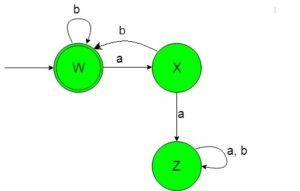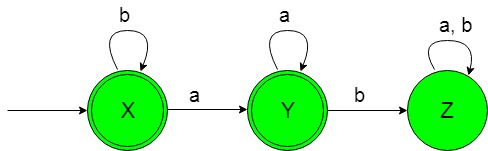# Designing Deterministic Finite Automata (Set 4)

Prerequisite: Designing finite automata, Designing Deterministic Finite Automata (Set 3)
In this article, we will see some designing of Deterministic Finite Automata (DFA).

Problem-1: Construction of a minimal DFA accepting set of strings over {a, b} in which every ‘a’ is followed by a ‘b’.
Explanation: The desired language will be like:

`L1 = {ε, ab, abab, abbbb, ababababab, ..............}`

Here as we can see that each string of the language containing ‘a’ just followed by ‘b’ but the below language is not accepted by this DFA because some of the string of the below language does not contain ‘a’ just followed by ‘b’.

`L2 = {ba, baab, bbaba, ..............}`

The state transition diagram of the language containing ‘a’ just followed by ‘b’ will be like:In the above DFA, state ‘W’ is the initial and final state too which on getting ‘b’ as the input it remains in the state of itself and on getting ‘a’ as the input it transit to a normal state ‘X’ which on getting ‘b’ as the input it transit to the final state ‘W’. The state ‘X’ on getting ‘a’ as input it transit to the dead state ‘Z’. The state ‘Z’ is called dead state because on getting any input it can not transit to the final state ever.

 `def` `stateW(n): ` `    ``if``(``len``(n)``=``=``0``): ` `        ``print``(``"Accepted"``) ` `    ``else``:   ` `         `  `        ``#if 'a' found  ` `        ``#call function stateX ` `        ``if` `(n[``0``]``=``=``'a'` `): ` `            ``stateX(n[``1``:]) ` `        ``#if 'b' found  ` `        ``#call function stateW     ` `        ``elif` `(n[``0``]``=``=``'b'` `): ` `            ``stateW(n[``1``:])     ` `         `  `def` `stateX(n): ` `    ``if``(``len``(n)``=``=``0``): ` `        ``print``(``"Not Accepted"``) ` `    ``else``:   ` `         `  `        ``#if 'a' found  ` `        ``#call function stateZ ` `        ``if` `(n[``0``]``=``=``'a'` `): ` `            ``stateZ(n[``1``:]) ` `         `  `        ``#if 'b' found  ` `        ``#call function stateW      ` `        ``elif` `(n[``0``]``=``=``'b'` `): ` `            ``stateW(n[``1``:])  ` `             `  `def` `stateZ(n): ` `    ``if``(``len``(n)``=``=``0``): ` `        ``print``(``"Not Accepted"``) ` `    ``else``: ` `         `  `         ``#if a or b found  ` `         ``#call stateZ ` `        ``if` `(n[``0``]``=``=``'a'` `or` `n[``0``]``=``=``'b'``): ` `            ``stateZ(n[``1``:])               ` ` `  ` `  ` `  `#take input ` `n``=``input``() ` ` `  `#call stateA ` `#to check the input ` `stateA(n) `

Problem-2: Construction of a minimal DFA accepting set of strings over {a, b} in which every ‘a’ is never be followed by ‘b’
Explanation: The desired language will be like:

`L1 = {ε, a, aa, aaaa, b, bba, bbbbba..............}`

Here as we can see that each string of the language containing ‘a’ is never be followed by ‘b’ but the below language is not accepted by this DFA because some of the string of the below language containing ‘a’ is followed by ‘b’.

`L2 = {ba, baab, bbaba, ..............}`

The state transition diagram of the language containing ‘a’ never be followed by ‘b’ will be like:In the above DFA, state ‘X’ is the initial and final state which on getting ‘b’ as the input it remains in the state of itself and on getting ‘a’ as input it transit to the final state ‘Y’ which on getting ‘a’ as the input it remains in the state of itself and on getting ‘b’ as input transit to the dead state ‘Z’. The state ‘Z’ is called dead state this is because it can not ever go to any of the final states.

 `def` `stateX(n): ` `    ``if``(``len``(n)``=``=``0``): ` `        ``print``(``"Accepted"``) ` `    ``else``:   ` `         `  `        ``#if 'a' found  ` `        ``#call function stateY ` `        ``if` `(n[``0``]``=``=``'a'` `): ` `            ``stateY(n[``1``:]) ` `        ``#if 'b' found  ` `        ``#call function stateX    ` `        ``elif` `(n[``0``]``=``=``'b'` `): ` `            ``stateX(n[``1``:])     ` `         `  `def` `stateY(n): ` `    ``if``(``len``(n)``=``=``0``): ` `        ``print``(``"Accepted"``) ` `    ``else``:   ` `         `  `        ``#if 'a' found  ` `        ``#call function stateZ ` `        ``if` `(n[``0``]``=``=``'a'` `): ` `            ``stateZ(n[``1``:]) ` `         `  `        ``#if 'b' found  ` `        ``#call function stateY    ` `        ``elif` `(n[``0``]``=``=``'b'` `): ` `            ``stateY(n[``1``:])  ` `             `  `def` `stateZ(n): ` `    ``if``(``len``(n)``=``=``0``): ` `        ``print``(``"Not Accepted"``) ` `    ``else``: ` `         `  `         ``#if a or b found  ` `         ``#call stateZ ` `        ``if` `(n[``0``]``=``=``'a'` `or` `n[``0``]``=``=``'b'``): ` `            ``stateZ(n[``1``:])               ` ` `  ` `  ` `  `#take input ` `n``=``input``() ` ` `  `#call stateA ` `#to check the input ` `stateA(n) `

Attention reader! Don’t stop learning now. Get hold of all the important CS Theory concepts for SDE interviews with the CS Theory Course at a student-friendly price and become industry ready.

My Personal Notes arrow_drop_upCheck out this Author's contributed articles.

If you like GeeksforGeeks and would like to contribute, you can also write an article using contribute.geeksforgeeks.org or mail your article to contribute@geeksforgeeks.org. See your article appearing on the GeeksforGeeks main page and help other Geeks.

Please Improve this article if you find anything incorrect by clicking on the "Improve Article" button below.